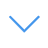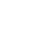# Math Puzzles CG

Math Puzzles CG is one of the Math Games that you can play on UGameZone.com for free. You need to calculate the number of each pattern according to the information provided in the game. Finally, the final answer to the question is obtained. This game can be as addition, subtraction, multiplication and division and requires strong logical thinking. Have fun.

Read MoreНажмите 【Esc】, чтобы выйти из полноэкранного режима.

# Math Puzzles CG

Math Puzzles CG is one of the Math Games that you can play on UGameZone.com for free. You need to calculate the number of each pattern according to the information provided in the game. Finally, the final answer to the question is obtained. This game can be as addition, subtraction, multiplication and division and requires strong logical thinking. Have fun.Для того, чтобы играть в эту игру, вам нужно разрешить Adobe Flash Player, чтобы работать в вашем браузере.

You can use UGameZone Flash Enabler plugin to enable Flash automatically. Or you can follow our instructions to enable Flash manually.

play automatically

## Game Ratings

81%

Пьесы 1040

Рейтинг 81%

Голосов 776

776
152
Category
Math Games
Теги
Описание

Math Puzzles CG is one of the Math Games that you can play on UGameZone.com for free. You need to calculate the number of each pattern according to the information provided in the game. Finally, the final answer to the question is obtained. This game can be as addition, subtraction, multiplication and division and requires strong logical thinking. Have fun.

Шаг 1
Шаг 2
Пожалуйста, включите Adobe Flash Player, чтобы играть в "Math Puzzles CG"

Включить Flash в настройках Chrome. нажмите « Блокировать сайты, на которых запущен Flash », переключитесь на « Сначала спросите вариант. Показано как следующее:Теперь нажмите и нажмите кнопку Разрешить в левом верхнем углу Chrome, чтобы начать игру. Показано как следующее: